# AP Macroeconomics : Flow of Funds

## Example Questions

### Example Question #1 : Real Interest Rate

The real interest rate can be approximated by the Fisher equation: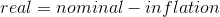What is the exact formula for the real interest rate?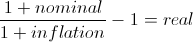The Fisher equation always gives the exact real interest rate.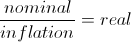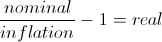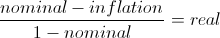Explanation:

The real interest rate is defined as the nominal appreciated value of assets divided by the new price level of the assets. The nominal appreciated value is simply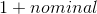, while the new price level is equal to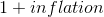. This gives the real appreciated value of assets. We then subtract 1 to get the real interest rate.

Example: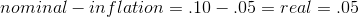(according to the Fisher equation)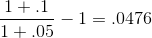The Fisher equation comes close, but is not actually correct.

### Example Question #1 : How To Find Level Of Investment

A consumer who discounts future payments at a rate of 10% per year will be willing to pay what amount for an asset that will pay $5000 in 3 years? Possible Answers:$4132.23

$6655$3756.57

$4545.45$5000

\$3756.57

Explanation:

The consumer's discount rate tells us what return on the investment the consumer must make every year in order to consider the asset worth buying. To determine the cost necessary to make the return worthwhile use the present discount formula: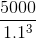### Example Question #1 : Net Exports

A depreciation in the value of a nation's currency will lead to what?

Higher exports

An increase in wages

An increase in tax revenue

Higher imports

Higher exports

Explanation:

A devaluation of a currency makes a nation's goods cost less to holders of foreign currency. Since the goods are now cheaper, there will be an increase in the quantity of goods demanded by holders of foreign currency. More foreigners buying goods means those goods must be shipped to them leading to an increase in exports.

### Example Question #1 : Net Exports

A trade surplus results from a country having __________.

net imports that exceed the total GDP

net imports that equal less than one third of total GDP

more net exports than net imports

net exports that equal more than one half of total GDP

more net imports than net exports